A+   a-

# Pre-Calculus and Trigonometry ReviewNOTE: This lesson is also available as executable worksheets on CoCalc. First, create an account and a project. Then you can copy these files to your project and start working right away. 01-review.ipynb (Jupyter Notebook) and 01-review.sagews (SageMath Worksheet).

This tutorial requires a working knowledge of functions and graphs. When we refer to f(x), we are referring to a function f whose values may change as x changes. For example, in this function: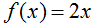f(x) is equal to 2 times x. We can evaluate the function when x is equal to ___ by replacing all occurrences of x with ___, then simplifying the resulting expression:and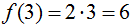To plot this function, we sketch a set of axes: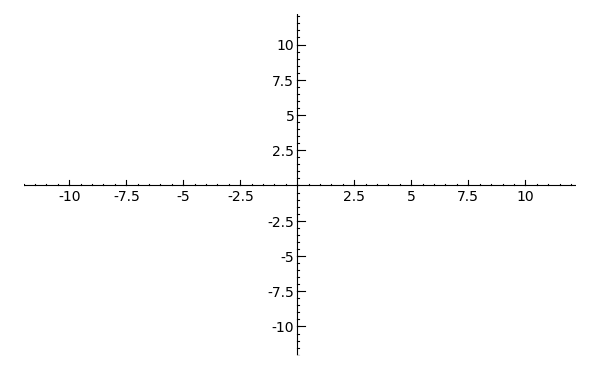Then we evaluate f(x) for a couple values of x (also known as a table of values) and plot the results according to their x-value -- moving along the horizontal x-axis -- and their y-value -- moving along the vertical y-axis -- and draw a line between them, like so: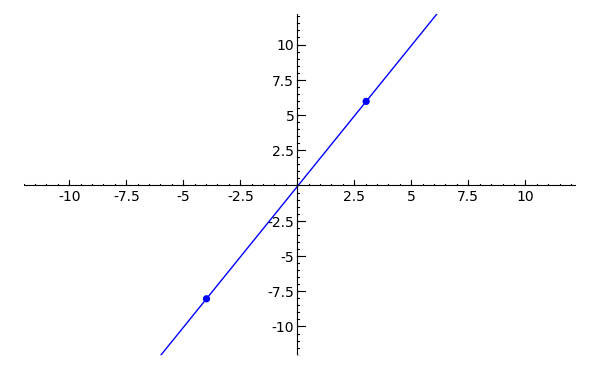As you can see, the graph intersects the x- and the y-axes at x=0 and y=0, respectively. To find the x-intercepts (if any) of a function g(x), replace g(x) with zero and solve for x. For example: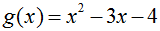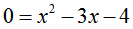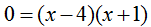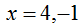Thus, g(x) has x-intercepts at x=-1 and x=4. Finding the function's y-intercept is easy; simply replace x with 0: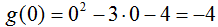Since a function can have at most one output for every input, a function can have at most one y-intercept. Conversely, since a function can have any number of inputs for one output, a function can have any number of x-intercepts.

## Transformations

Given a function f(x), if,

then f(x) will be:

• stretched vertically by a factor of |m|
• compressed horizontally by a factor of |n|
• displaced horizontally to the right by a units
• displaced vertically upward by b units
• If m is negative, f(x) will be inverted over the y-axis
• If n is negative, f(x) will be inverted over the x-axis
• The sign of a and b affects the direction of the function's displacement.

To apply this, try manipulating the function f(x) = 2*x. If you have the SageMath notebook open, start a new worksheet and copy the following code into the first cell. Manipulate m, n, a, and b (and/or f(x) itself), then evaluate the code to see how the graph changes.

```m = -2
n = 1/10
a = 1
b = 2

def f(x):
return 2*x

plot(m*f(n*(x-a))+b, x, -10, 10).show(ymin=-10, ymax=10)
```
Toggle Explanation Toggle Line Numbers

1-4) Set variable m, n, a and b
6-7) Define a function f(x) that equals 2*x
9) Plot f(x) with various transformations

# Trigonometry

Trigonometry is the math of triangles -- or as you have probably experienced, the math of triangles inscribed in circles. The following images from Wikipedia are visual representations of the trigonometric functions and the unit circle, respectively.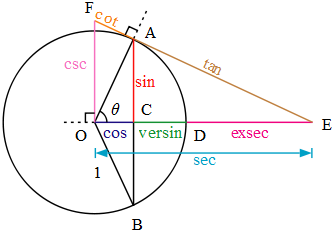This calculus tutorial requires no knowledge of versin or exsec, but you should be familiar with cosine, sine, and tangent, and have passing knowledge of cosecant, secant, and cotangent. Remember SOHCAHTOA (pronounced 'soak-uh-toe-uh'): Sine is Opposite over Hypotenuse, Cosine is Adjacent over Hypotenuse, and Tangent is Opposite over Adjacent.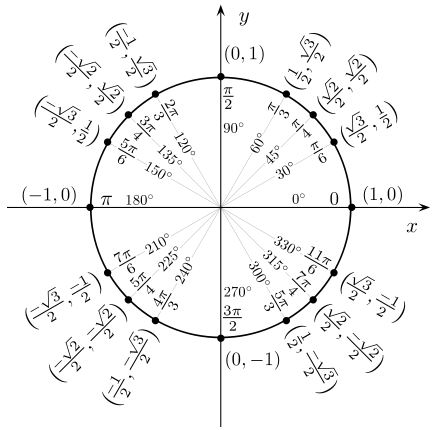In the pictured unit circle, the coordinate pairs describe the cosine of the angle (comma) the sine of the angle. It shows that the cosine of pi over 3 is one-half, while the sine of pi over three is root three over two, and so forth. The units involving pi are known as radians: one would say, 'an angle of three pi over two radians', which is also equivalent to two hundred seventy degrees.

For this or any exploration of calculus, there are some basic trigonometric identities with which you should be familiar. In approximate order of importance, they are: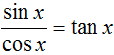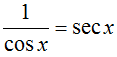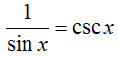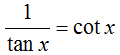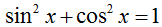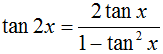To refresh your memory, here are some graphs of trigonometric functions: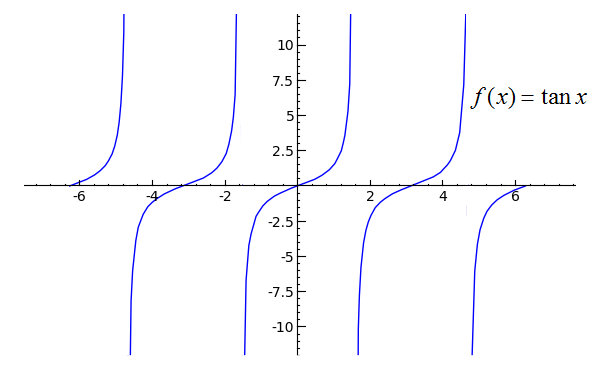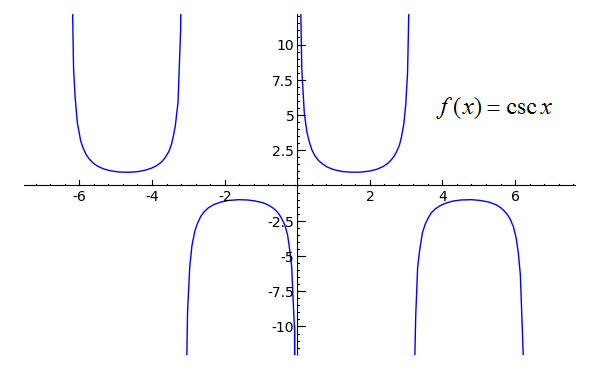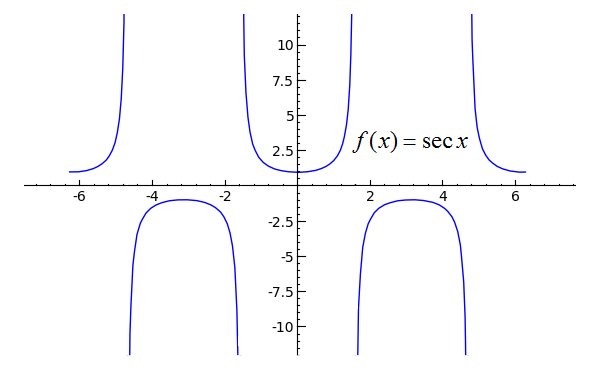The period of sine, cosine, secant, and cosecant is two pi, while the period of tangent and cotangent is pi. A function's period is the horizontal width of its repeating section. Since the sine function has a period of two pi, the interval [0, 2π] is identical to [2π, 4π]. Similarly, sine's interval [3π/8, 19π/8] is identical to [35π/8, 51π/8].

Well, that's it for the review. I tried to keep it fairly straightforward, but if there is something you would like added/clarified, please let me know. That aside, it's time to go forth and learn some calculus!